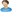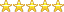Home»Economics» An econometric analysis of the effects of monetary policy on the Nigerian economy

# An econometric analysis of the effects of monetary policy on the Nigerian economy

Department: Economics
By:mattkings

Project ID: 741
Rating:(5.0) votes: 2
Rate this project
Price:₦1000
Abstract
This study aimed at analyzing through econometric methodology the effects of monetary policy in Nigeria economy. To meet the above objective, output growth was chosen as the dependent variable while real exchange rate, real interest rate, and inflation were chosen as the independent variable. The ordinary least square was used in the regression estimation. From the empirical result, we realized that the entire explanatory variables are insignificant in the t-test, but in f-test, we rejected the null hypothesis and conclude that the slope coefficient are not simultaneously equal to zero. We realize from the battery test that there is cointegration between the explanatory band the dependent variables since its level of stationarity are the same. The policy implication of the result is that if monetary and banking policies are effectively applied, it will be consistent with determining the level of output growth in the economy ...
Preview +
Other Economics project topics and materials you might be interested in